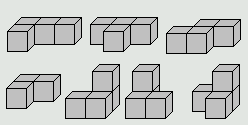### Problem Statement

Soma is a three dimensional puzzle invented by Piet Hein. You have seven pieces which are formed by joining cubes at their faces. (They are all of the non-convex shapes that can be so formed with four or fewer cubes). Six of the pieces are formed from four cubes and one is formed by three cubes. There are 27 cubes total. The pieces can be described by the following arrays which show how high cubes are stacked in each grid cell, and are also shown in the picture below.

```1 1 1   1 1 1   0 1 1
1 0 0   0 1 0   1 1 0

1 1   0 2   2 0   1 2
1 0   1 1   1 1   0 1
```The pieces can be translated and rotated into any orientation, to build larger shapes, but can not be reflected (as in a mirror) or disassembled. Pieces may touch, but not intersect. Each piece is used exactly once in a solution.

Given pattern (a shape composed of 27 cubes, not necessarily connected), try to arrange the seven soma pieces into the same shape as pattern. In other words, you are constructing pattern using the seven pieces. Return the number of distinct solutions for pattern.

What is meant by "distinct":

In a valid solution each cube in pattern will be occupied by a cube from exactly one of the seven pieces. You could assign a number between 1 and 7 inclusive to each pattern cube to indicate which piece occupies that pattern cube. Two solutions are distinct if this assignment is different at one or more pattern cubes. Thus removing a piece which has rotational symmetry, rotating it, and putting it back "in the same place" does not produce a new distinct solution. But a rearrangement of some or all of the pieces which is equivalent to rotating or reflecting the entire pattern (assuming pattern has such symmetry) is considered distinct by this definition. For example the pattern, "21112", can be constructed in exactly two distinct ways using the pieces "211" and "12". The distinct ways are "211"-"12" and "21"-"112".

The pattern will be specified in a String[] similar to the arrays showing the individual pieces above. Each character indicates how many cubes are stacked at that location, starting from a common plane at a height of zero.

For example:

```{"333",
"333",
"333"}
```

specifies a 3x3x3 cube which is possible to construct with the seven soma pieces in 11520 distinct (as defined above) ways, so return 11520.

### Definition

 Class: Soma Method: letMeCountTheWays Parameters: String[] Returns: int Method signature: int letMeCountTheWays(String[] pattern) (be sure your method is public)

### Notes

-If no rotational symmetries are involved, there are 24 possible orthogonal orientations resulting from rotations in three dimensions. Visualize the 3x2 "L" shaped piece (which has no rotational symmetry). The top (long end) of the "L" can point in six directions x,y,z,-x,-y,-z. For each of those, the short leg of the "L" can point in one of four directions. 6 x 4 = 24
-Each of the other six pieces does have some rotational symmetry, and thus fewer possible distinct orientations.
-The rotation of a point about a line passing through the origin can be calculated using a single, vector by matrix, multiplication: [x y z]*M=[rx ry rz] where M is a 3 by 3 matrix, [x y z] is the original point and [rx ry rz] is the rotated point.
-Vector by matrix multiplication is defined as: for(i) { r[i]=0 ; for(j) { r[i]+=p[j]*M[j][i] } } where p is the original point and r is the rotated point.
-The matrix for 90 degree rotation about the x axis is: {{1,0,0},{0,0,1},{0,-1,0}}
-The matrix for 90 degree rotation about the y axis is: {{0,0,-1},{0,1,0},{1,0,0}}
-The matrix for 90 degree rotation about the z axis is: {{0,1,0},{-1,0,0},{0,0,1}}
-The easiest way to generate all possible orthogonal orientations is to rotate about the x axis (0,90,180 or 270 degrees), then about the y axis (0,90,180 or 270 degrees) then about the z axis (0,90,180,270 degrees). That is 64 combinations of rotations (4x4x4). Try all 64 and throw away those that produce duplicate results.
-Sequences of rotations in three dimensions are non-commutative. The order in which you apply the rotations matters.
-There are 240 fundamental patterns to form the cube, proven by the great mathematician, Prof. John H. Conway. 240 x 24 rotations x 2 (for mirror reflection) = 11520. There are 96 ways to rotate individual pieces in each of the 11520 "distinct" ways. 11520 x 96 = 1105920 which is the "over a million ways to build a cube" number the manufacturer uses.

### Constraints

-pattern will contain between 2 and 27 elements, inclusive.
-Each element of pattern will consist of between 2 and 27 characters, inclusive.
-Each element of pattern will have the same number of characters.
-Each character in each element of pattern will be a digit between '0' and '9' inclusive.
-The sum of all the digits in pattern will be exactly equal to 27.

### Examples

0)

 ```{"333", "333", "333"}```
`Returns: 11520`
 The cube.
1)

 ```{"345", "234", "123"}```
`Returns: 2800`
 The crystal.
2)

 ```{"3330000", "0033300", "0000333"}```
`Returns: 28`
 The wall.
3)

 ```{"21111111", "21111111", "21111111"}```
`Returns: 0`
 The chase lounge, impossible.
4)

 ```{"67", "77"}```
`Returns: 1520`
 The tower.
5)

 ```{"010000000000000000000000000", "110000000000000000000000000", "000000000000000000000000000", "000000000000000000000000000", "000000000000000000000000000", "000000000000000000000000000", "000000000000000000000000000", "000000000000000000000000000", "000000000000000000000000000", "000000000000000000000000000", "000000000000000000000000000", "000000000000000000000000000", "000000000000000000000000000", "000000000000000000000000000", "000000000000000000000000000", "000000000000000000000000000", "000000000000000000000000000", "000000000000000000000000000", "000000000000000000000000000", "000000000000000000000000000", "000000000000000000000000000", "000000000000000000000000000", "000000000000000000000000000", "000000000000000000000000022", "000000000000000000000000022", "000000000000000000000002222", "000000000000000000000002222"}```
`Returns: 76`
 Replication.
6)

 ```{"11100110001", "01001100111", "00000000000", "20002012011", "11011001001"}```
`Returns: 1`
 Disjoint.
7)

 ```{"121", "222", "121", "121", "333"}```
`Returns: 78`
 The monument.
8)

 ```{"020", "010", "010", "020", "343", "353"}```
`Returns: 42`
 The gallows.

#### Problem url:

http://www.topcoder.com/stat?c=problem_statement&pm=2824

#### Problem stats url:

http://www.topcoder.com/tc?module=ProblemDetail&rd=5073&pm=2824

Rustyoldman

#### Testers:

lbackstrom , brett1479 , schveiguy

#### Problem categories:

Brute Force, Geometry